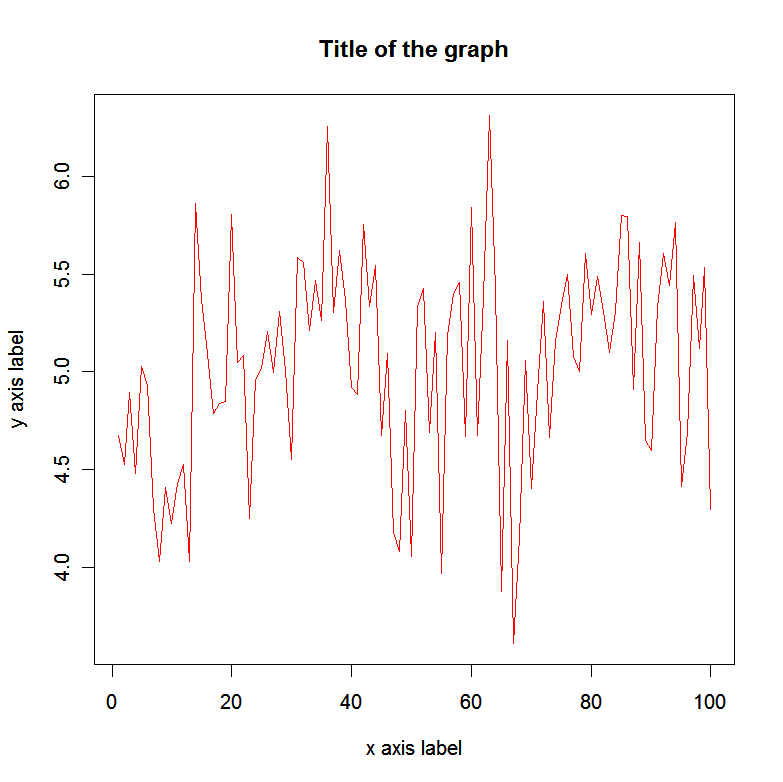Friday, August 3, 2012

How to add data points on plots in R

In R if you want to add a custom data point on the graph, e.g on the xy position (40,6), with the color 40 ("burlywood3"), and with the line width 3, type:
points(40,6, col=14, lwd=3)

If we follow up the graph made in the previous post:This would be the resulting graph:If we want to add multiple points type e.g. the following:
points(c(10,20,30,40,50,60,70,80,90),c(4,4,4,5,5,5,6,6,6), col=14, lwd=3)

If you want to connect these points into a line:
points(c(10,20,30,40,50,60,70,80,90),c(4,4,4,5,5,5,6,6,6), col=14, lwd=3, type="l")